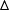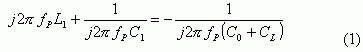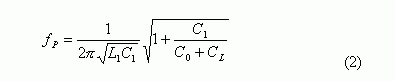# Modeling of Quartz Crystal

### Abstract

This application note, describes a method for modeling a crystal, with loading capacitance.

Clock generator (CLK gen) designers can use a crystal model to easily determine the loading capacitance for a required frequency. Circuit and system designer can use the model to simulate the behavior of crystal oscillators.

To model a crystal, we use a simple circuit to measure the oscillation frequencies under different loading capacitance. Then, we employ the crystal circuit model to interpolate the measured data to determine the parameters in the circuit model.

### Introduction

Quartz crystals have been widely used in clock generators and synthesizers to generate accurate reference frequencies. The most cost-effective crystals on the market have a frequency range of 30kHz to 30MHz, with 50-100 ppm accuracy in the 0°C to 70°C temperature range.

To use or design a crystal oscillator appropriately, it is important to know the crystal's behavior under its loaded condition. In this application note, we will introduce a method for modeling a crystal with loading capacitance and show applications of the model.

### Test Setup

The test setup is shown in Figure 1.In the setup, A1 and A2 are inverters; CL1 and CL2 are the loading capacitors. During the test, CL1 = CL2 and the value varies from 5pf to 59pf with the inverter supply voltage VCC = 3.1V and VCC = 2.3V. The crystal in the test has a nominal frequency of 27MHz at load capacitance of 14pF. It should be noted that the actual loading capacitance to the crystal equals CL1 || CL2 plus the parasitic capacitance of board and the terminals of the inverters.

### Test Results

Table 1 and 2 present the two sets of measurements for VCC=3.1V and 2.3V, respectively.

 CL1, CL2 (pf) 5 8 12 15 18 20 22 FOUT (MHz) 27.0141 27.0083 27.0058 27.0039 27.0019 27.0013 27.0004FOUT (ppm) 523 308 216 146 70 48 14 CL1, CL2 (pf) 24 27 33 39 45 50 59 FOUT (MHz) 26.9995 26.9986 26.9969 26.9959 26.9948 26.9942 26.9934FOUT (ppm) -17 -53 -116 -151 -193 -213 -244
 CL1, CL2 (pf) 5 8 12 15 18 20 22 FOUT (MHz) 27.0132 27.0078 27.0054 27.0036 27.0016 27.0011 27.0002FOUT (ppm) 489 288 200 133 59 39 6 CL1, CL2 (pf) 24 27 33 39 45 50 59 FOUT (MHz) 26.9993 26.9984 26.9967 26.9958 26.9947 26.9942 26.9933FOUT (ppm) -24 -60 -121 -156 -197 -217 -249

The two measured data sets are also plotted in Figure 2.From the data measurement, we have the following observations:

1. Loading capacitors can change the crystal's oscillation frequency considerably. The result shows that the total crystal variation range can reach as high as 750ppm for the crystal under test.
2. The frequency variation is also dependent on VCC. Lower supply voltage reduces the frequency. This may be due to the changes of inverter input and output capacitance caused by the change of supply voltage. The resistor R2 in Figure 1 has an effect on reducing such a voltage dependency. But the value of the resistor cannot be too large; otherwise it will make the oscillator hard to start.
3. Based on the data, the crystal frequency is much more sensitive to small loading capacitance. This implies that in application of crystal oscillator, we should use a crystal requiring relative large load capacitance for its nominal frequency.
4. The resistor R1 in Fig. 1 helps the oscillator to start. Also the inverter's characters affect the oscillator's performance. High-speed inverters should be used. If the inverter's speed is not high enough, the oscillation may not start.

### Modeling of Quartz Crystal

In the oscillator or VCXO design, the crystal model is required. A commonly adopted crystal resonator model [1-2] is depicted as Figure 3.Next, we will use the measured data given in Tables 1 and 2 to determine the values of the components in Figure 3. According to , in most crystal oscillator applications, the oscillation frequency is the parallel frequency of the resonator in Fig 3. Define CL = CL1 || CL2 and fp to be the oscillation frequency, we can have the impedance equation written as:Solve the equation for fP yielding,DefineWe then can rewrite Eq. (2) in a vector form as:With Eq. (4) we can run the least-square estimation directly to estimate fs, C1, L1, and C0. It should be noted that the value of R1 is usually given by the crystal datasheet. For the crystal used in our test, R1 = 40Ω. To estimate C0, at a given supply voltage, vary the value of C0 to get the best least-square fit to the measured data sets, assuming that the effect of VCC is only on the value of C0. The estimated component's values of the model in Figure 3 are listed as follows:In Figure 4, it shows the output frequency curve calculated by Eq. (2) with the estimated component values verse the measured frequencies. The root of mean square (RMS) of the interpolation is 14ppm for the case of VCC = 3.1V and 13ppm for VCC = 2.3V.### Summary

In this application note, we have shown a test setup for measuring the oscillation frequency of a quartz crystal and a method to estimate the parameters of the crystal's resonator model. Real measurements reveal how oscillation frequency varies with loading capacitance.

We introduced a numerical approach based on measured data, to estimate the parameters of the crystal's resonator circuit model. As shown in Figure 4, the model fits the measured data closely with a root of mean square error (RMS) of 13-14ppm. Clock generator designers can use the model to determine the shunt capacitor values for a required frequency. System and circuit design engineers can use the model for system behavior simulations.

### References

1. Norman Bujanos, "Choosing the Right Crystal for Your Oscillator", EDN, Feb., 1998, pp. 66-70.
2. James Northcutt, "Specifying Crystal for Use in VCXOs and TCXOs for Wireless Designs", Wireless Design and Development, Feb., 1998.